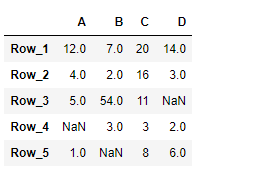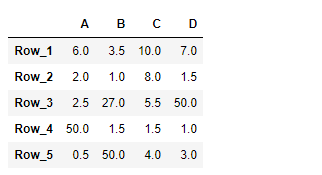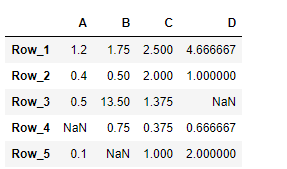# Python | Pandas DataFrame.truediv

Pandas DataFrame is a two-dimensional size-mutable, potentially heterogeneous tabular data structure with labeled axes (rows and columns). Arithmetic operations align on both row and column labels. It can be thought of as a dict-like container for Series objects. This is the primary data structure of the Pandas.

Pandas` DataFrame.truediv()` function perform the floating division of dataframe and other, element-wise. It is equivalent to `dataframe / other`, but with support to substitute a fill_value for missing data in one of the inputs.

Syntax: DataFrame.truediv(other, axis=’columns’, level=None, fill_value=None)

Parameter :
other : scalar, sequence, Series, or DataFrame
axis : {0 or ‘index’, 1 or ‘columns’}
level : Broadcast across a level, matching Index values on the passed MultiIndex level.
fill_value : Fill existing missing (NaN) values, and any new element needed for successful DataFrame alignment.

Returns : Result of the arithmetic operation.

Example #1 : Use `DataFrame.truediv()` function to perform division of the given dataframe with a scalar element-wise. Also fill 100 at the place of all the missing values.

 `# importing pandas as pd ` `import` `pandas as pd ` ` `  `# Creating the DataFrame ` `df ``=` `pd.DataFrame({``"A"``:[``12``, ``4``, ``5``, ``None``, ``1``],  ` `                   ``"B"``:[``7``, ``2``, ``54``, ``3``, ``None``],  ` `                   ``"C"``:[``20``, ``16``, ``11``, ``3``, ``8``],  ` `                   ``"D"``:[``14``, ``3``, ``None``, ``2``, ``6``]})  ` ` `  `# Create the index ` `index_ ``=` `[``'Row_1'``, ``'Row_2'``, ``'Row_3'``, ``'Row_4'``, ``'Row_5'``] ` ` `  `# Set the index ` `df.index ``=` `index_ ` ` `  `# Print the DataFrame ` `print``(df) `

Output :Now we will use `DataFrame.truediv()` function to perform division of the given dataframe by 2, element-wise. We are going to fill 100 at the place of all the missing values in this dataframe.

 `# divide by 2 element-wise ` `# fill 100 at the place of missing values ` `result ``=` `df.truediv(other ``=` `2``, fill_value ``=` `100``) ` ` `  `# Print the result ` `print``(result) `

Output :As we can see in the output, the `DataFrame.truediv()` function has successfully performed the division of the given dataframe by a scalar.

Example #2 : Use `DataFrame.truediv()` function to perform the division of the given dataframe using a list.

 `# importing pandas as pd ` `import` `pandas as pd ` ` `  `# Creating the DataFrame ` `df ``=` `pd.DataFrame({``"A"``:[``12``, ``4``, ``5``, ``None``, ``1``],  ` `                   ``"B"``:[``7``, ``2``, ``54``, ``3``, ``None``],  ` `                   ``"C"``:[``20``, ``16``, ``11``, ``3``, ``8``],  ` `                   ``"D"``:[``14``, ``3``, ``None``, ``2``, ``6``]})  ` ` `  `# Create the index ` `index_ ``=` `[``'Row_1'``, ``'Row_2'``, ``'Row_3'``, ``'Row_4'``, ``'Row_5'``] ` ` `  `# Set the index ` `df.index ``=` `index_ ` ` `  `# Print the DataFrame ` `print``(df) `

Output :Now we will use `DataFrame.truediv()` function to perform division of the given dataframe using a list.

 `# divide using a list ` `# across the column axis ` `result ``=` `df.truediv(other ``=` `[``10``, ``4``, ``8``, ``3``], axis ``=` `1``) ` ` `  `# Print the result ` `print``(result) `

Output :As we can see in the output, the `DataFrame.truediv()` function has successfully performed the division of the given dataframe by a list.

My Personal Notes arrow_drop_upCheck out this Author's contributed articles.

If you like GeeksforGeeks and would like to contribute, you can also write an article using contribute.geeksforgeeks.org or mail your article to contribute@geeksforgeeks.org. See your article appearing on the GeeksforGeeks main page and help other Geeks.

Please Improve this article if you find anything incorrect by clicking on the "Improve Article" button below.

Article Tags :

Be the First to upvote.

Please write to us at contribute@geeksforgeeks.org to report any issue with the above content.